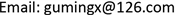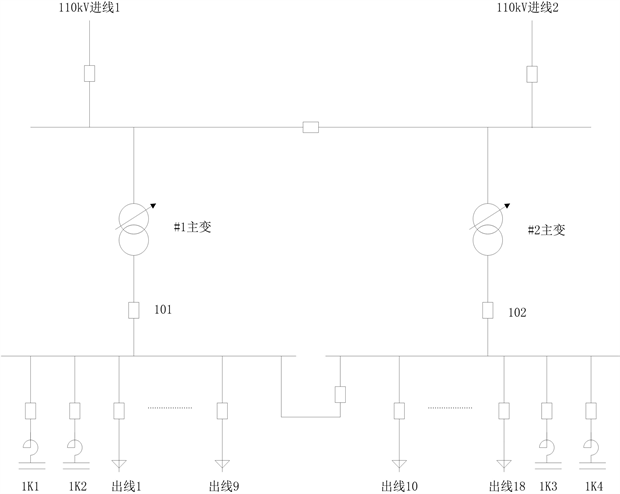﻿ 一起110 kV变电站并联电容器装置谐波放大的分析与对策 The Analysis and Solutions for the Harmonic Amplification Occurred from Shunt Capacitor Devices in the 110 kV Substation

Transmission and Distribution Engineering and Technology
Vol. 08  No. 04 ( 2019 ), Article ID: 33584 , 10 pages
10.12677/TDET.2019.84016

The Analysis and Solutions for the Harmonic Amplification Occurred from Shunt Capacitor Devices in the 110 kV Substation

Mingxing Gu1, Xi Zhang1, Xiaolian Zhang2

1Changshu Power Supply Company, State Grid Jiangsu Electric Power Company, Changshu Jiangsu

2School of Electric Power Engineering, Nanjing Institute of Technology, Nanjing JiangsuReceived: Nov. 27th, 2019; accepted: Dec. 17th, 2019; published: Dec. 24th, 2019ABSTRACT

Based on the analysis of the harmonic amplification phenomena, after the shunt capacitors of a 110 kV substation been turned into operation, the effect of harmonics amplification on the current power system is analyzed quantitatively. The paper investigates the rationality of capacitor reactance in series, and verifies with the field test data. The improved measures are put forward, which provide reference for how to select the reactance rate of shunt capacitor reasonably in practical application.

Keywords:Substation, Shunt Capacitor, Harmonic Amplification, Reactance Rate1国网江苏省电力公司常熟市供电分公司，江苏 常熟

2南京工程学院电力工程学院，江苏 南京1. 引言

2. 某110 kV变电站谐波超标问题

2.1. 用户运行中的电能质量问题

2019年9月2日，供电公司收到江苏省太湖地区水利工程管理所相关材料，客户反映在投入10 kV高压电容补偿柜后有异常，怀疑电网背景谐波注入影响。

2.2. 变电站运行概况

2.2.1. 变电站主接线

2.2.2. 并联电容器配置Figure 1. The primary wiring diagram of a 110 kV substation

2.3. 现场谐波测试情况Table 1. The table of distortion rate and main characteristics harmonic voltage containing rate on 10 kV I bus

1K1、1K2电容器组均切除时，101#1主变开关侧5次谐波电流超过国标限值(查明为115大义线上华宇针织印染和昌盛印染5次谐波电流严重超标导致，已请营销督促用户进行谐波治理)。当投入1K1或1K2电容器装置时，101#1主变开关侧5次、7次谐波电流均出现放大现象。101#1主变开关侧各相谐波电流变化情况如表2所示。Table 2. The table of main characteristics harmonic current in 101#1 switch side of transformerTable 3. The table of main characteristics harmonic voltage on the capacitor circuitTable 4. The table of main characteristics harmonic current in the capacitor circuit

3. 谐波放大的成因分析

3.1. 谐波电流放大的基本原理Figure 2. Simplified analysis diagram of power supply system

$s={X}_{s}/{X}_{C}$$k={X}_{K}/{X}_{C}$ 分别为以 ${X}_{C}$ 为基值的系统电抗率和电抗器电抗率，则主系统和电容器的谐波电流可以表示为

${I}_{Ch}=\frac{{X}_{sh}}{{X}_{sh}+{X}_{Kh}-{X}_{Ch}}{I}_{h}=\frac{h{X}_{s}}{h{X}_{s}+h{X}_{K}-{X}_{C}/h}{I}_{h}=\frac{s}{s+k-1/{h}^{2}}{I}_{h}$ (1)

${I}_{sh}=\frac{{X}_{Kh}-{X}_{Ch}}{{X}_{sh}+{X}_{Kh}-{X}_{Ch}}{I}_{h}=\frac{h{X}_{K}-{X}_{C}/h}{h{X}_{s}+h{X}_{K}-{X}_{C}/h}{I}_{h}=\frac{k-1/{h}^{2}}{s+k-1/{h}^{2}}{I}_{h}$ (2)

1) 在 ${h}_{0}=1/\sqrt{s+k}$ 时， ${I}_{Ch}=\infty$${I}_{sh}=\infty$，主系统和电容器的谐波电流均被无限放大(实际上由于电阻的存在， ${I}_{Ch}$${I}_{sh}$ 是有限大值)，为谐振状态；

2) 在 ${h}_{k}=1/\sqrt{k}$ 时， ${I}_{Ch}={I}_{h}$${I}_{sh}=0$，电容器完全吸收谐波，为全滤波状态；

3) 在 ${h}_{1}=1/\sqrt{2s+k}$ 时， ${I}_{Ch}=-{I}_{h}$${I}_{sh}=2{I}_{h}$，为谐波严重放大的第一临界状态；

4) 在 ${h}_{2}=1/\sqrt{s/2+k}$ 时， ${I}_{Ch}=2{I}_{h}$${I}_{sh}=-{I}_{h}$，为谐波严重放大的第二临界状态；

3.2. 参数计算和验证

${X}_{s}=\frac{{U}_{c}^{2}}{{S}_{oc}}=\frac{{10.5}^{2}}{256}=0.43\text{\hspace{0.17em}}\Omega$Table 5. Typical conditions of harmonic current amplification

${X}_{C1}=\frac{{U}^{2}×1000}{{S}_{N1}/3}=\frac{{\left(11/\sqrt{3}\right)}^{2}×1000}{4800/3}=25.21\text{\hspace{0.17em}}\Omega$

${X}_{C2}=\frac{{U}^{2}×1000}{{S}_{N2}/3}=\frac{{\left(11/\sqrt{3}\right)}^{2}×1000}{6000/3}=20.17\text{\hspace{0.17em}}\Omega$

${X}_{K}=\frac{{Q}_{K}}{{I}_{K}^{2}}=\frac{10×{10}^{3}}{{400}^{2}}=0.063\text{\hspace{0.17em}}\Omega$

${s}_{1}={X}_{s}/{X}_{C1}=0.43/25.21=1.7%$${k}_{1}={X}_{K}/{X}_{C1}=0.063/25.21=0.25%$

${s}_{2}={X}_{s}/{X}_{C2}=0.43/20.17=2.13%$${k}_{2}={X}_{K}/{X}_{C2}=0.063/20.17=0.31%$Table 6. Typical conditions of harmonic current correspond to harmonic numberTable 7. The main characteristic harmonic amplification in theoryTable 8. The main characteristic harmonic amplification under actual test

4. 对策与措施

4.1. 并联电容器装置串联电抗率的调整

${{h}^{\prime }}_{0}=\frac{1}{\sqrt{s+{k}^{\prime }}}>3,\text{\hspace{0.17em}}{{h}^{\prime }}_{k}=\frac{1}{\sqrt{{k}^{\prime }}}<5$ (3)Table 9. When k = 5%, typical conditions of harmonic current correspond to harmonic number

4.2. 谐波电压的放大校验

$|{F}_{sh}|=|\frac{k{h}^{2}-1}{{h}^{2}\left(s+k\right)-1}|$ (4)Table 10. The check table of harmonic voltage amplification times on 10 kV bus

4.3. 电容器过电流与过电压校验

$|{F}_{Ch}|=|\frac{{h}^{2}s}{{h}^{2}\left(s+k\right)-1}|$ (5)

${I}_{Ch}=|{F}_{Ch}|{I}_{h}$

${U}_{Ch}={I}_{Ch}{X}_{C}/h$

$\sqrt{{I}_{C1}^{2}+\sum {I}_{Ch}^{2}}\le 1.15{I}_{CN}$ (6)

${U}_{C1}+\sum {U}_{Ch}\le 1.2{U}_{CN}$ (7)

${U}_{C1}={U}_{C2}=\frac{1.05{U}_{c}}{\sqrt{3}S\left(1-k\right)}=\frac{1.05×10.5×1000}{\sqrt{3}×1×\left(1-0.05\right)}=6700\text{\hspace{0.17em}}\text{V}$

${X}_{C1}=25.21\text{\hspace{0.17em}}\Omega$${X}_{C2}=20.17\text{\hspace{0.17em}}\Omega$，可得1K1、1K2电容器基波电流分别为：

${I}_{C1}=\frac{{U}_{C1}}{{X}_{C1}}=\frac{6700}{25.21}=266\text{\hspace{0.17em}}\text{A}$${I}_{C2}=\frac{{U}_{C2}}{{X}_{C2}}=\frac{6700}{20.17}=332\text{\hspace{0.17em}}\text{A}$Table 11. The main characteristics harmonic component of the capacitor circuit

5. 结语

The Analysis and Solutions for the Harmonic Amplification Occurred from Shunt Capacitor Devices in the 110 kV Substation[J]. 输配电工程与技术, 2019, 08(04): 131-140. https://doi.org/10.12677/TDET.2019.84016

1. 1. 姜松青. 变电站用高压并联电容器成套装置[J]. 山东工业技术, 2017(5): 281.

2. 2. 国家电网公司. 并联电容器装置技术及应用[M]. 北京: 中国电力出版社, 2011.

3. 3. 胡谆, 杨猛, 吕文韬. 某变电站20kV并联电容器投运后谐波测试分析[J]. 机电信息, 2017(12): 1-2.

4. 4. 江卫中, 徐青龙, 翟国柱, 等. 110kV变电站并联电容器装置谐波谐振分析与对策[J]. 供用电, 2017, 34(11): 73-77.

5. 5. 沈东方, 张华. 10kV无功自动补偿装置的谐波放大分析[J]. 电力电容器与无功补偿, 2009, 30(1): 12-15.

6. 6. 郭昆丽, 田海霞, 王建波, 等. 某变电站10kV并联电容器故障分析及对策[J]. 广东电力, 2017, 30(3): 58-63.

7. 7. 曾海涛, 欧雄坚, 胡少强. 并联电容器谐波放大引起的10kV线路断路器跳闸事故的分析[J]. 机电工程技术, 2015, 44(1): 108-111.

8. 8. 国家能源局. 电能质量技术监督规程: DL/T 1053-2017[S]. 北京: 中国电力出版社, 2017.

9. 9. 国家技术监督局. 电能质量, 公用电网谐波: GB/T 14549-1993[S]. 北京: 中国标准出版社, 1994.

10. 10. 国家质量监督检验检疫总局. 电磁兼容, 试验和测量技术, 供电系统及所连设备谐波、间谐波的测量和测量仪器导则: GB/T 17626.7-2017[S]. 北京: 中国标准出版社, 2017.

11. 11. 肖湘宁等. 电能质量分析与控制[M]. 北京: 中国电力出版社, 2010.

12. 12. 中国电力企业联合会. 并联电容器装置设计规范: GB 50227-2017[S]. 北京: 中华人民共和国住房和城乡建设部, 2017.

13. 13. 周胜军, 林海雪. 并联电容器装置参数的工程选择计算[J]. 供用电, 2009, 26(4): 9-14, 51.

14. 14. 陶梅, 江钧祥. 串联电抗器及其电抗率的选取[J]. 电力电容器与无功补偿, 2010, 31(3): 58-61.

15. 15. 邓朴, 刘晓波, 皮显松, 等. 电容器串联电抗消除谐振的最大值最小优化[J]. 电力系统保护与控制, 2016, 44(10): 71-78.